CAT  >  Test: Quadratic Equations- 4

# Test: Quadratic Equations- 4

Test Description

## 15 Questions MCQ Test Quantitative Aptitude (Quant) | Test: Quadratic Equations- 4

Test: Quadratic Equations- 4 for CAT 2022 is part of Quantitative Aptitude (Quant) preparation. The Test: Quadratic Equations- 4 questions and answers have been prepared according to the CAT exam syllabus.The Test: Quadratic Equations- 4 MCQs are made for CAT 2022 Exam. Find important definitions, questions, notes, meanings, examples, exercises, MCQs and online tests for Test: Quadratic Equations- 4 below.
Solutions of Test: Quadratic Equations- 4 questions in English are available as part of our Quantitative Aptitude (Quant) for CAT & Test: Quadratic Equations- 4 solutions in Hindi for Quantitative Aptitude (Quant) course. Download more important topics, notes, lectures and mock test series for CAT Exam by signing up for free. Attempt Test: Quadratic Equations- 4 | 15 questions in 15 minutes | Mock test for CAT preparation | Free important questions MCQ to study Quantitative Aptitude (Quant) for CAT Exam | Download free PDF with solutions
 1 Crore+ students have signed up on EduRev. Have you?
Test: Quadratic Equations- 4 - Question 1

### Find the maximum value of the expression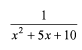Detailed Solution for Test: Quadratic Equations- 4 - Question 1

For the given expression to be a maximum, the denominator should be minimized. (Since, the function in the denominator has imaginary roots and is always positive), x2 + 5x + 10 will be minimized at x = -2.5 and its minimum values at x = -2.5 is 3.75.
Hence, required answer = 1/3.75 =4/15.

Test: Quadratic Equations- 4 - Question 2

### Find the minimum value of the expression (p +1/p); p > 0.

Detailed Solution for Test: Quadratic Equations- 4 - Question 2

The minimum value of (p + 1/p) is at p = 1. The value is 2.

Test: Quadratic Equations- 4 - Question 3

### The expression a2 + ab + b2 is _________for a < 0, b < 0

Detailed Solution for Test: Quadratic Equations- 4 - Question 3

For a, b negative the given expression will always be positive since, a2, b2 and ab are all positive.

Test: Quadratic Equations- 4 - Question 4

Find the roots of the quadratic equation: x2 + 2x - 15 = 0?​

Detailed Solution for Test: Quadratic Equations- 4 - Question 4

x2 + 5x - 3x - 15 = 0
x(x + 5) - 3(x + 5) = 0
(x - 3)(x + 5) = 0
=> x = 3 or x = -5.

Test: Quadratic Equations- 4 - Question 5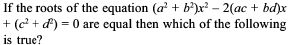Detailed Solution for Test: Quadratic Equations- 4 - Question 5

Solve this by assuming each option to be true and then check whether the given expression has equal roots for the option under check.

Thus, if we check for option (b). ad = be.
We assume a = 6, d = 4 b = 12 c = 2 (any set o f values that satisfies ad = bc).
Then (a2 + b2) x2 - 2(ac + bc) x + (c2 + d2) = 0

180 x2- 120 x +20 = 0.
We can see that this has equal roots. Thus, option (b) is a possible answer. The same way if we check for a, e and d we see that none of them gives us equal roots and can be rejected.

Test: Quadratic Equations- 4 - Question 6

Two numbers a and b are such that the quadratic equation ax2 + 3x + 2b = 0 has - 6 as the sum and the product of the roots. Find a + b.

Detailed Solution for Test: Quadratic Equations- 4 - Question 6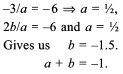Test: Quadratic Equations- 4 - Question 7

Find the value of the expression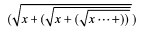Detailed Solution for Test: Quadratic Equations- 4 - Question 7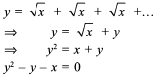Solving quadratically, we have option (b) as the root of this equation.

Test: Quadratic Equations- 4 - Question 8

If the roots of the equation (a2 + b2) x2 - 2b(a + c) x + (b2 + c2) = 0 are equal then a, b, c, are in

Detailed Solution for Test: Quadratic Equations- 4 - Question 8

Solve by assuming values of a, b, and c in AP, GP and HP to check which satisfies the condition.

Test: Quadratic Equations- 4 - Question 9

If x2 + ax + b leaves the same remainder 5 when divided by x - 1 or x + 1 then the values of a and b are respectively

Detailed Solution for Test: Quadratic Equations- 4 - Question 9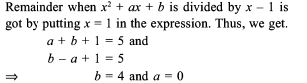Test: Quadratic Equations- 4 - Question 10

Read the data given below and it solve the questions based on.
If α and β? are roots of the equation x2 + x - 7 = 0 then.

Find α2 + β2

Detailed Solution for Test: Quadratic Equations- 4 - Question 10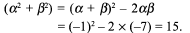Test: Quadratic Equations- 4 - Question 11

For what values o f c in the equation 2x2 - (c3 + 8c - l)x + c2 - 4c = 0 the roots of the equation would be opposite in signs?

Detailed Solution for Test: Quadratic Equations- 4 - Question 11

For the roots to be opposite in sign, the product should be negative.

(c2 - 4x)/2 < 0 ⇒ 0 < c < 4 .

Test: Quadratic Equations- 4 - Question 12

The expression x2 + kx + 9 becomes positive for what values of k (given that x is real)?

Detailed Solution for Test: Quadratic Equations- 4 - Question 12

The roots should be imaginary for the expression to be positive i.e.

k2 - 36 < 0

thus - 6 < k < 6 or |k| < 6.

Test: Quadratic Equations- 4 - Question 13

In the Maths Olympiad of 2020 at Animal Planet, two representatives from the donkey’s side, while solving a quadratic equation, committed the following mistakes: (i) One of them made a mistake in the constant term and got the roots as 5 and 9. (ii) Another one committed an error in the coefficient of x and he got the roots as 12 and 4.But in the meantime, they realised that they are wrong and they managed to get it right jointly. Find the quadratic equation.

Detailed Solution for Test: Quadratic Equations- 4 - Question 13

From (i) we have sum o f roots = 1 4 and from (ii) we have product of roots = 48.
Option (c) is correct.

Test: Quadratic Equations- 4 - Question 14

For what value of b and c would the equation x2 + bx + c = 0 have roots equal to b and c.

Detailed Solution for Test: Quadratic Equations- 4 - Question 14

Solve using options. It can be seen that at b = 0 and c = 0 the condition is satisfied. It is also satisfied at b = 1 and c = - 2 .

Test: Quadratic Equations- 4 - Question 15

A journey between Mumbai and Pune (192 km apart) takes two hours less by a car than by a truck. Determine the average speed of the car if the average speed of the truck is 16 km/h less than the car.

Detailed Solution for Test: Quadratic Equations- 4 - Question 15

Solve using options, If the car’s speed is 48 kmph, the bus’s speed would be 32 kmph. The car would take 4 hours and the bus 6 hours.A journey between Mumbai and Pune (192 km apart) takes two hours less by a car than by a truck. Determine the average speed of the car if the average speed of the truck is 16 km/h less than the car. (a) 48 km/h (b) 64 km/h (c) 16 km/h (d) 24 km/h

## Quantitative Aptitude (Quant)

163 videos|152 docs|131 tests
 Use Code STAYHOME200 and get INR 200 additional OFF Use Coupon Code
Information about Test: Quadratic Equations- 4 Page
In this test you can find the Exam questions for Test: Quadratic Equations- 4 solved & explained in the simplest way possible. Besides giving Questions and answers for Test: Quadratic Equations- 4, EduRev gives you an ample number of Online tests for practice

## Quantitative Aptitude (Quant)

163 videos|152 docs|131 tests

### How to Prepare for CAT

Read our guide to prepare for CAT which is created by Toppers & the best Teachers